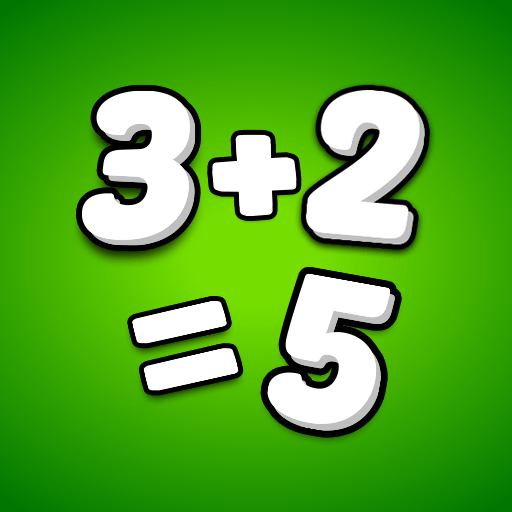# Math Game: Math Games For Kids

4.8

##### About Math Game: Math Games For Kids
Math games are a great way to challenge your child and develop logical thinking through learning games for kids: learn and have fun at the same time. Math games and practice worksheets are designed especially for preschoolers and early years to learn mathematics game and enhance their Math skills. Learning math for kids through puzzles, learning games for kids, pictures and fun tools makes math interesting for your child through math game. They are able to learn addition, subtraction, multiplication, math games multiplication tables, sequencing and number counting with the help of our mathematics games kids learning app as part of free kids games. All this makes this kids math game a very good version of educational games for kids. So be it addition games exercise, subtraction game working or multiplication tables from multiplication games, this math games will help you cover all topic.

Math games for kids is designed as a real addition to educational games keeping in mind the cognitive ability of 3 to 8 years old for best learning. We aim to make math champs through kids learning games! Maths addition, subtraction, multiplication and division techniques, easy methods and strategies shared makes a child’s mind sharp and quick at solving sums in math games. Solving arithmetic & mathematics games problems are the basics for higher level maths and all kids should be able to solve simple addition and subtraction by the age of 5 years with math games. This math game will provide you training from addition games, multiplication games and subtraction game, all within one educational. Practicing in number game is the only way to sharpen math skills and math games brain training unseen in usual kids learning apps. Our math for kids games, worksheets and fun math game puzzles make math extremely easy for kids. Kids are motivated to do more without getting bored in educational game. Maths app and games makes math enjoyable for children of all age groups.

Math games is FREE to play and is aimed to improve math for kids in the following segment:

- Become logical thinkers through math games
- Enhance math memory through colorful designs
- Solve math brain training puzzles
- Subtraction game sums and calculation techniques
- Mathematics multiplication games techniques
- Easy to learn math tables
- Use easy methods to solve challenging math questions
- Different number game difficulty level adjusted according to age groups, while considering step by step growth and progress of kids in mathematics games offline
- Worksheets developed by subject specialist of math game enhance practice & brain training

Enjoy ➕ addition, ➖ subtraction, ➗ division and ✖️ multiplication exercises in learning games for kids. Explore the Math games fun in this educational app and make your child a Math Champ!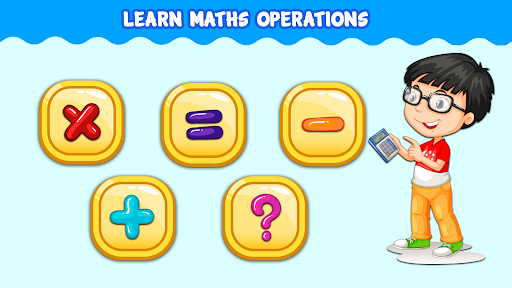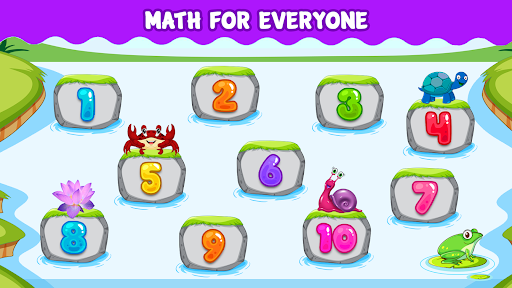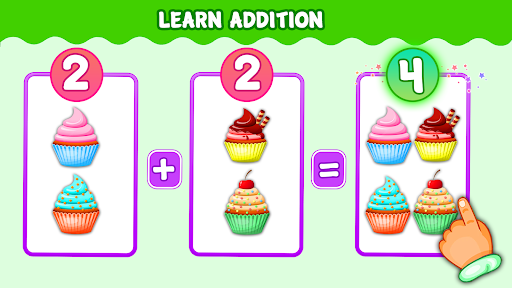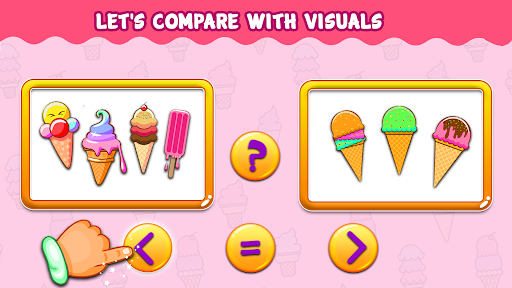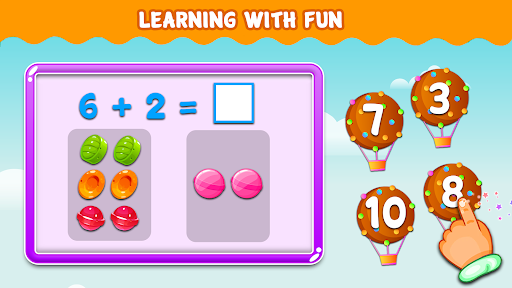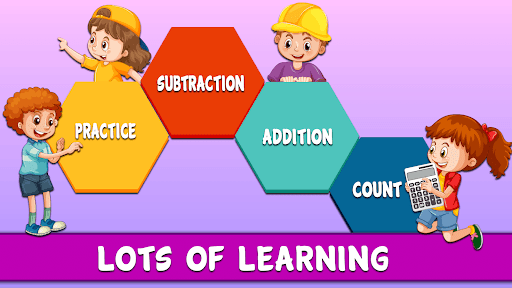##### Recent Updates - Version 1.0.1
• Dear Parents,
We have came up with another learning game for your kids, in this Math Game your kids will be able to learn the following:
- Become logical thinkers through math games
- Enhance memory through colorful designs
- Solve puzzles